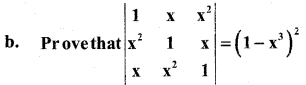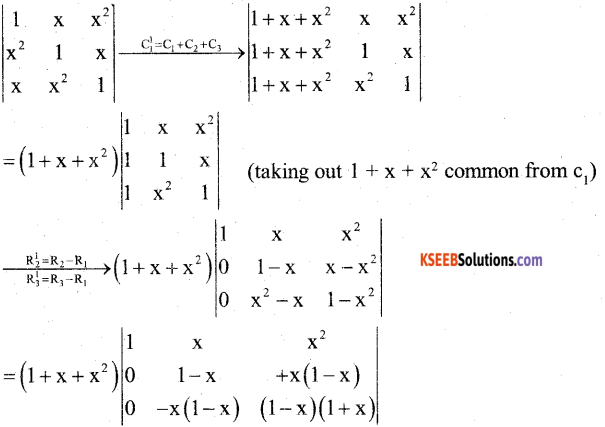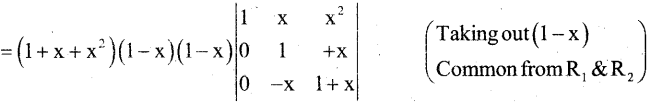# 2nd PUC Maths Previous Year Question Paper June 2019

Students can Download 2nd PUC Maths Previous Year Question Paper June 2019, Karnataka 2nd PUC Maths Model Question Papers with Answers helps you to revise the complete Karnataka State Board Syllabus and score more marks in your examinations.

## Karnataka 2nd PUC Maths Previous Year Question Paper June 2019

Time: 3 Hrs 15 Min
Max. Marks: 100

Part – A

Answer ALL the following questions: (10 × 1 = 10)

Question 1.
Let * be the binary operation on N given.by a * b = LCM of a and b, ∀ a ? b ∈ N.
Given a * b = LCM of a and b ∀ a, b ∈ N 5*7 = 35

Question 2.
Find the principal value of cot-1$$\left(-\frac{1}{\sqrt{3}}\right)$$
Cot-1$$\left(-\frac{1}{\sqrt{3}}\right)$$ = π – Cot-1$$\left(-\frac{1}{\sqrt{3}}\right)$$
[∵ cot-1(-x) = π – cot-1(x)]
= π – $$\frac{\pi}{3}$$
∴ cot-1 $$\left(\frac{-1}{\sqrt{3}}\right)=\frac{2 \pi}{3}$$

Question 3.
If A = [aij] where elements are given by aij = $$\frac { 1 }{ 2 }$$|-3i + j| construct 2 × 2 matrix.
Given aij = $$\frac { 1 }{ 2 }$$ |-3i + j|
a11 = $$\frac { 1 }{ 2 }$$|-3(1) + 1|= $$\frac { 1 }{ 2 }$$|-2| = 1
a12 = $$\frac { 1 }{ 2 }$$ |-3(1) + 2| = $$\frac { 1 }{ 2 }$$|-l| = $$\frac { 1 }{ 2 }$$
a21 = $$\frac { 1 }{ 2 }$$ |-3(2) + 2| = $$\frac { 1 }{ 2 }$$ |-5| = $$\frac { 5 }{ 2 }$$
a22 = $$\frac { 1 }{ 2 }$$ |-3(2) + 2| = $$\frac { 1 }{ 2 }$$ |-4| = 2

Question 4.
Find a value of x for which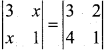3 – x2 = 3 – 8 ⇒ x2 = 8 ⇒ x = ±2$$\sqrt{2}$$Question 5.
If y = cos-1 (ex), find $$\frac{d y}{d x}$$.
y = cos-1 (ex) (diff wrt x)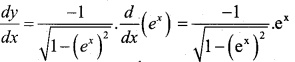Question 6.
Find $$\int \sec ^{2}(7-4 x) d x$$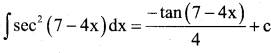Question 7.
If â = $$\frac{1}{\sqrt{14}}$$ (2î + 3ĵ + k̂), then write the directions cosines of â.
â = $$\frac{1}{\sqrt{14}}$$ (2î + 3ĵ + k̂)
∴ Directions cosines are :
$$\frac{2}{\sqrt{14}}, \frac{3}{\sqrt{14}}, \frac{1}{\sqrt{14}}$$

Question 8.
Find the intercepts cut off by the plane 2x + y – z = 5
2x + y – z = 5 …..(1) ÷ 5
$$\frac{2 x}{5}+\frac{y}{5}-\frac{z}{5}=1$$
or $$\frac{x}{(5 / 2)}+\frac{y}{5}+\frac{z}{(-5)}=1$$
∴ x – intercept = $$\frac { 5 }{ 2 }$$ ; y – intercept = 5, z – intercept = -5

Question 9.
Define feasible region in a linear programming problem.
Feasible region is defined as the common region determined by all the constraints including non – negative constraints x ≥ 0, y ≥ 0 of a linear programming problem is called as Feasible region.

Question 10.
If P(A) = $$\frac { 3 }{ 5 }$$ and P(B) = $$\frac { 1 }{ 5 }$$ find P(A ∩ B), where A and B are independent events.
P(A) = $$\frac { 3 }{ 5 }$$; P(B) = $$\frac { 1 }{ 5 }$$
P(A ∩ B) = P(A).P(B)
[Since A and B are independent events]
= $$\frac{3}{5} \cdot \frac{1}{5}$$ = $$\frac { 3 }{ 25 }$$

Part-B

Answer any TEN questions: (10 x 2 = 20)

Question 11.
Find g.f and f.g, if (x) = 8x3 and g(x) = x1/3
Given
f(x) = 8x3 ,g(x)= x1/3
fog (x) = f(g(x))
= f(x1/3) =8(x3)1/3 =8x
gof (x) = g(f(x)) = g(8x3)
= (8x3)1/3 = 2xQuestion 12.
Prove that tan-1 $$\frac { 2 }{ 11 }$$ + tan-1 $$\frac { 7 }{ 24 }$$ = tan-1 $$\frac { 1 }{ 2 }$$
Consider LHS : tan-1 ($$\frac { 2 }{ 11 }$$) + tan-1 ($$\frac { 7 }{ 24 }$$)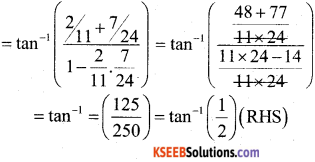Question 13.
Write cot-1 $$\left(\frac{1}{\sqrt{x^{2}-1}}\right)$$ ,x > 1 in the simplest form.
Let x = sec q ⇒ q = sec-1 x.
Now cot-1 $$\left(\frac{1}{\sqrt{x^{2}-1}}\right)$$ = cot-1 $$\left(\frac{1}{\sqrt{\sec ^{2} \theta-1}}\right)$$
= cot-1 $$\left(\frac{1}{\tan \theta}\right)$$ = cot-1(cot θ) = θ = sec-1 x.

Question 14.
Find the area of the triangle with vertices (3,8), (-4, 2) and (5, 1) using determinants.
Area of triangle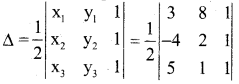= $$\frac { 1 }{ 2 }$$ [3(2 – 1) -8(-4 -5) + 1(-4 -10)]
= $$\frac { 1 }{ 2 }$$ [3 + 72 -14] = $$\frac { 61 }{ 2 }$$sq.units

Question 15.
Find $$\frac{d y}{d x}$$ if x2 + xy + y2 = 100.
x2 + xy + y2 = 100 (diff wrt x)
2x + x.$$\frac{d y}{d x}$$ + y.1 + 2y$$\frac{d y}{d x}$$ = 0
x.$$\frac{d y}{d x}$$ + 2y$$\frac{d y}{d x}$$ = 2x – y
$$\frac{d y}{d x}$$(x + 2y) = -(2x + y)
$$\therefore \frac{d y}{d x}=\frac{-(2 x+y)}{x+2 y}$$Question 16.
Find $$\frac{d y}{d x}$$ if y = (logx)cosx.
Let y = (log x)cos x
⇒ log y = cos x log (log x)
⇒ $$\frac{d }{d x}$$logy = $$\frac{d }{d x}$$cosx log(logx)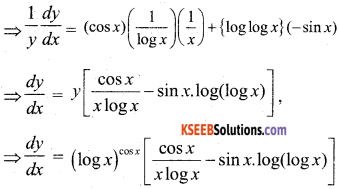Question 17.
Find the interval in which the function f given f(x) = 2x2 – 3x is strictly increasing.
f(x) = 2x2 – 3x
diff wrt x
f1 (x) = 4x – 3
f1 (x) = 0 ⇒ 4x – 3 = 0
or 4x = 3
⇒ x = 3/4
∴ Point x = 3/4 divides the real line into two disjoint intervals (- ∞, 3/4) and (3/4, ∞) In the interval (3/4, ∞), f1(x) = 4x – 3 > 0
∴ f is strictly increasing in (3/4, ∞)

Question 18.
Find $$\int \frac{\left(x^{4}-x\right)^{\frac{1}{4}}}{x^{5}} d x$$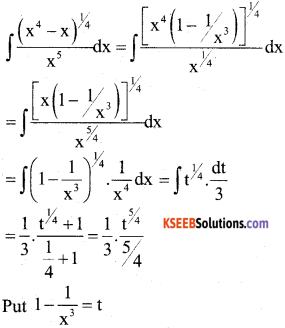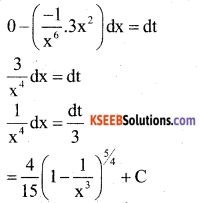Question 19.
Integrate x sec2 x with respect to x.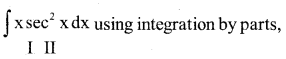$$\int x \sec ^{2} x d x=x \int \sec ^{2} x d x-\int\left(\int \sec ^{2} x\right) \cdot \frac{d}{d x}(x) \cdot d x$$
= x. tan x – $$\int \tan x \cdot 1 \cdot d x$$
= x . tanx – log |sec x| + c

Question 20.
Find the order and degree (if defined) of the differential equation :
y111 – y2 + e3 = 0
Order = 3, Degree = not defined.

Question 21.
If $$\overrightarrow{\mathrm{a}}$$ is a unit vector and $$(\vec{x}-\vec{a}) \cdot(\vec{x}+\vec{a})$$ = 8, then find $$|\overrightarrow{\mathrm{x}}|$$ .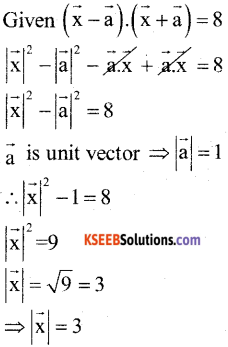Question 22.
Find the area of the parallelogram whose adjacent sides are given by the vectors $$\overrightarrow{\mathrm{a}}$$ = 3î + ĵ + 4 k̂ and $$\overrightarrow{\mathrm{b}}$$ = î – ĵ + k̂
Area of parallelogram = $$|\overrightarrow{\mathrm{a}} \times \overrightarrow{\mathrm{b}}|$$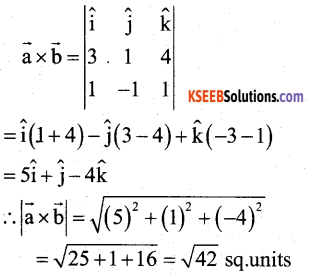Question 23.
Find the angle between the pair of lines $$\frac{x+3}{3}=\frac{y-1}{5}=\frac{z+3}{4}$$ and $$\frac{x+1}{1}=\frac{y-1}{1}=\frac{z-5}{2}$$
Given
$$\frac{x+3}{3}=\frac{y-1}{5}=\frac{z+3}{4}$$
Here $$\overrightarrow{\mathrm{b}}_{1}$$ = 3î + 5ĵ + 4k̂
and $$\frac{x+1}{1}=\frac{y-1}{1}=\frac{z-5}{2}$$ ⇒ î + ĵ + 2k̂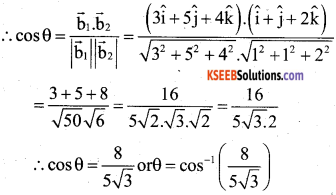Question 24.
The probability distribution of random variable X is as follows :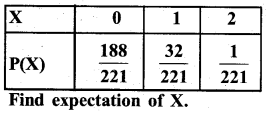Here E(X) = Σxi P(xi)
$$=0 \times \frac{188}{221}+1 \times \frac{32}{221}+2 \times \frac{1}{221}=\frac{32+2}{221}=\frac{34}{221}$$

Part-C

Answer any TEN questions: (10 × 3 = 30)

Question 25.
Determine whether the relation R in the set A = {1, 2, 3, …., 13, 14} defined as R = {(x,y); 3x – y = 0} is reflexive, symmetric and transitive.
Given A = {1,2,3, ….,13, 14}
R = {(x, y) : 3x – y =0}
∴ R= {1,3), (2, 6), (3, 9), (4, 12)}
Here (1, 1) ∉ R, (2, 2) ∉ R
R is not reflexive
Here (1, 3) ∈ R but (3, 1) ∉ R
∴ R is not symmetric
Here (1,3) and (3, 9)6 ∈ R but (1,9) ∉ R
∴ R is not transitive
∴ R is not an equivalence relation.

Question 26.
Solve for x, if tan-1 2x + tan-13x = $$\frac{\pi}{4}$$, x > 0
Given tan-1(2x) + tan-1(3x) = $$\frac{\pi}{4}$$
tan-1 $$\left(\frac{2 x+3 x}{1-2 x \cdot 3 x}\right)$$ = $$\frac{\pi}{4}$$
$$\frac{5 x}{1-6 x^{2}}$$ = tan $$\frac{\pi}{4}$$
⇒ $$\frac{5 x}{1-6 x^{2}}$$ = 1 ⇒ 5x = 1 – 6x2
∴ 6x2 + 5x – 1 = 0
6x2 + 6x – x – 1 =0
6x(x+1) -1(x+1) = 0 or (x+1) (6x – 1)= 0
⇒ x = -1 or x = $$\frac { 1 }{ 6 }$$Question 27.
By using elementary transformations, find the inverse of the matrix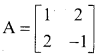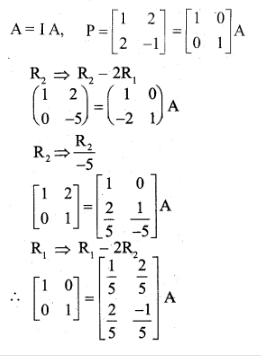Question 28.
If x = a(θ + sinθ), y = a( 1 – cosθ) then show that $$\frac{d y}{d x}$$ = tan $$\left(\frac{\theta}{2}\right)$$.
we have
$$\frac{d x}{d \theta}$$ = a(1 + cosθ), $$\frac{d y}{d \theta}$$ = a(sin θ)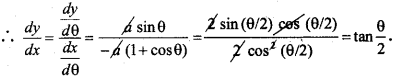Question 29.
Verify mean value theorem for the function f(x) = x2 – 4x – 3 in the interval [1, 4].
f(x) = x2 – 4x – 3 interval [a, b] = [1,4]
Here f is a polynomial function hence continuous in [1, 4] and differentiable in (1,4)
f(x) = x2 – 4x – 3 (diff wrt x)
∴ f1(x) = 2x – 4 1
Also f(1) = 12 – 4 – 3 = -6
f(4) = 42 – 4(4) – 3 = -3
∴ From MVT ∃ c ∈ (a,b) such that
f1 (c) = $$\frac{f(b)-f(a)}{b-a}=\frac{-3-(-6)}{4-1}$$
f1 (c) = $$\frac { 3 }{ 3 }$$ = 1
f1 (c) = 2c – 4
∴ 2c – 4 = 1
or 2c = 5
c = $$\frac { 5 }{ 2 }$$ ∈ (1, 4)Question 30.
Find the point, at which the tangent to the curve y = $$\sqrt{4 x-3}-1$$ has its slope $$\frac { 2 }{ 3 }$$
y = $$\sqrt{4 x-3}-1$$ (diff wrt x)
$$\frac{d y}{d x}=\frac{1}{2 \sqrt{4 x-3}} \cdot 4$$
Given slope of tangent = $$\frac{d y}{d x}=\frac{2}{3}$$
∴ $$\frac{2}{3} =\frac{1}{2 \sqrt{4 x-3}} .4$$
$$\frac{4}{3} =\frac{4}{\sqrt{4 x-3}} \Rightarrow \sqrt{4 x-3}=3$$
$$\sqrt{4 x-3} = 3$$ (squaring)
4x – 3 = 9
4x = 12
0r x = 3
∴ y = $$\sqrt{4(3)-3-1}$$
= $$\sqrt{9}$$ – 1 = 3 – 1 = 2
∴ point = (3, 2)

Question 31.
Find $$\int \frac{x}{(x+1)(x+2)} d x$$.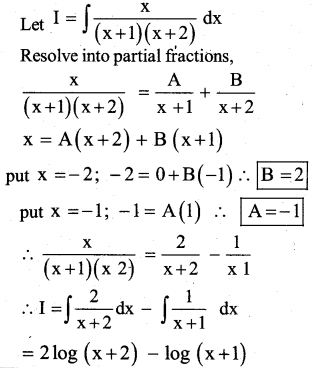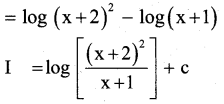Question 32.
Evaluate $$\int_{0}^{2}\left(x^{2}+1\right) d x$$. as a limit of a sum.
$$\int_{0}^{2}\left(x^{2}+1\right) d x$$ Here a = 0 ; b = 2
f (x) = x2 + 1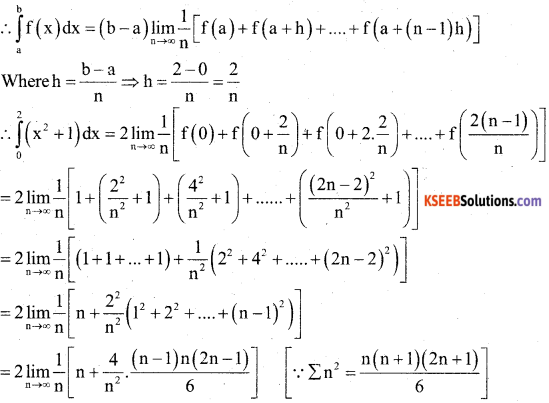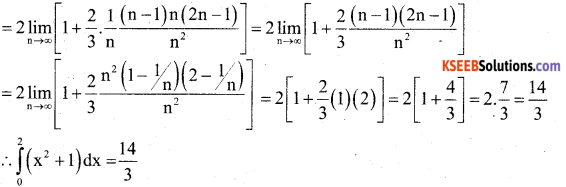Question 33.
Find the area of the region bounded by y2 = 9x, x = 2, x = 4 and the x – axis in the first quadrant.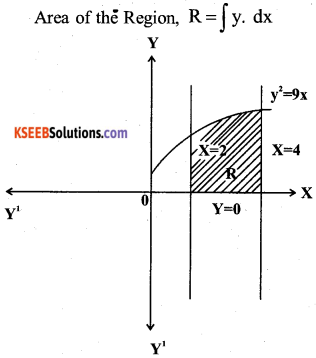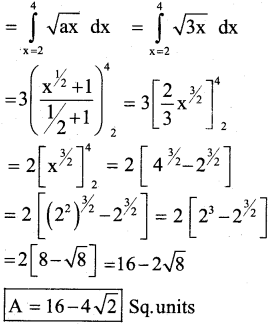Question 34.
If y = ae3x + be-2x represents family of curves, where a and b are arbitrary constants. Form the differential equation.
Given, y = ae3x + be-2x …. (1)
y’ = (ae3x)3 – (be-2x) 2
y’ = 3a e3x – 2be-2x …. (2)
From (1) and (2)
y = ae3x + be-2x × 3
y’ = 3a e3x – 2b e-2x × 1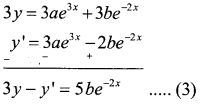Diff
⇒ 3y’ – y” = 5be-2x
3y’ – y”= -10be-2x …. (4)
From (3) & (4)
3y’ – y” = (-2) (5be-2x)
3y’ – y” = -2[3y – y’]
3y’- y” = -6y + 2y’
y” – y’ – 6y= 0.Question 35.
Show that the position vector of the point P, which divides the line joining the points A and B having position vectors $$\overrightarrow{\mathrm{a}}$$ and $$\overrightarrow{\mathrm{b}}$$ internally in the ratio
$$\mathbf{m}: \mathbf{n} \text { is } \frac{\mathbf{m} \overrightarrow{\mathbf{b}}+\mathbf{n} \overrightarrow{\mathbf{a}}}{\mathbf{m}+\mathbf{n}}$$
Here P divides AB internally in the ratio m : n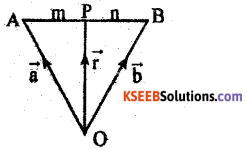∴ p divides $$\overrightarrow{\mathrm{AB}}$$ such that
$$\frac{\mathrm{AP}}{\mathrm{PB}}=\frac{\mathrm{m}}{\mathrm{n}}$$ or n$$\overrightarrow{\mathrm{AP}}$$ = m$$\overrightarrow{\mathrm{PB}}$$
Where m and n are positive scalars
Here $$\overrightarrow{\mathrm{r}}, \overrightarrow{\mathrm{a}}, \overrightarrow{\mathrm{b}}$$ are position vectors of $$\overrightarrow{\mathrm{OP}}$$
$$\overrightarrow{\mathrm{OA}}$$ an $$\overrightarrow{\mathrm{OB}}$$ respectively
∴ From the figure we have,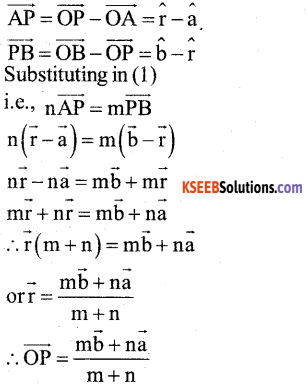Question 36.
Prove that $$[\overrightarrow{\mathbf{a}}+\overrightarrow{\mathbf{b}}, \overrightarrow{\mathbf{b}}+\overrightarrow{\mathbf{c}}, \overrightarrow{\mathbf{c}}+\overrightarrow{\mathbf{a}}]$$ = 2$$[\vec{a}, \vec{b}, \vec{c}]$$
LHS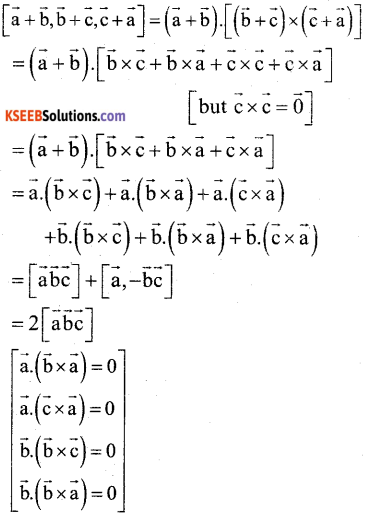Question 37.
Find the shortest distance between the lines $$\overrightarrow{\mathbf{r}}=\hat{\mathbf{i}}+\hat{\mathbf{j}}+\lambda(2 \hat{\mathbf{i}}-\hat{\mathbf{j}}+\hat{\mathbf{k}})$$ $$\overrightarrow{\mathbf{r}}=2 \hat{\mathbf{i}}+\hat{\mathbf{j}}-\hat{\mathbf{k}}+\mu(3 \hat{\mathbf{i}}-5 \hat{\mathbf{j}}+2 \hat{\mathbf{k}})$$
2nd PUC Maths Previous Year Question Paper June 2019 27

Question 38.
Bag I contains 3 red and 4 black balls. White Bag II contains 5 red and 6 black balls. One ball is drawn at random from one of the bags and it is found to be red. Find
Let A be the event of choosing the bag I
B be the event of choosing the bag II
and C be the event of drawing a red ball
∴ P(A) = P(B) = $$\frac { 1 }{ 2 }$$
Also p(C/A) = $$\frac { 3 }{ 7 }$$ and P(C/B) = $$\frac { 5 }{ 11 }$$
∴ P(C)=P(A).P(C/A) +P(B).P(C/B)
$$=\frac{1}{2} \times \frac{3}{7}+\frac{1}{2} \times \frac{5}{11}=\frac{3}{14}+\frac{5}{22}=\frac{33+35}{154}$$
P(C) = $$\frac { 68 }{ 154 }$$
By Baye’s Theorem,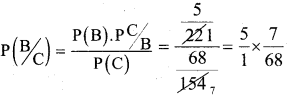P(B/C) = $$\frac { 35 }{ 68 }$$Part-D

Answer any SIX questions: (6 × 5 = 30)

Question 39.
Prove that the function f = R → R defined by f(x) = 4x + 3, is invertible and find the inverse of f.
Let f(x1) = f(x2)= 4x1 + 3 ⇒ 4x2 + 3
⇒ 4x1 = 4x2 ⇒ x1 = x1
f is onto.
Let y ∈ R∃ x ∈ R such that f(x) = y
4x + 3 = y $$x=\frac{y-3}{4} \in R$$
∴ f is onto ∴ f is bijection
∴ f is invertible
Define a function g : R → R by
g(y) = $$\frac{y-3}{4}$$
(fog) (y) = f(g(y)) = f$$\frac{y-3}{4}$$
= 4$$\frac{y-3}{4}$$+3 = y – 3 + 3 = y ∴ (fog) (y) = y fog = I
(gof) (x) = g(f(x)) = g (4x + 3)
$$=\frac{4 x+3-3}{4}=\frac{4 x}{4}=x$$
∴ (gof) (x) = x ∴gof = I
∴ f-1(y) = g(y) = $$\frac{y-3}{4}$$ ∀ y ∈ R

Question 40.
If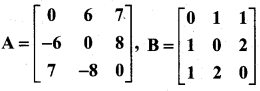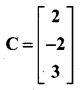Calculate AC, BC and (A + B)C.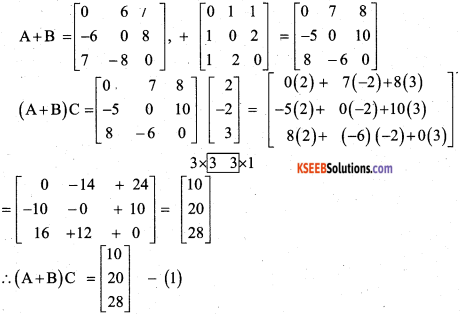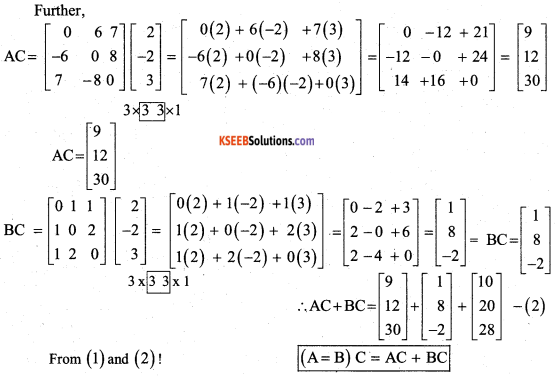Question 41.
Solve the following system of linear equations by matrix method:
3x – 2y + 3z = 8
2x + y – z = 1
4x – 3y + 2z = 4
The system of equations can be written in the form A X = B where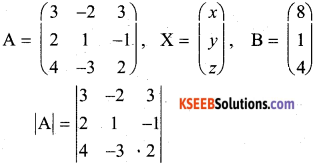|A| = 3 (2 – 3) + 2(4 + 4) + 3 (-6 -4) = – 17
Hence, A is non singular and so its inverse exists. Now
A11 = -1, A12 = -8 A13 = -10
A21 = -5, A22 = -6 A23 = +1
A31 = -1, A32 = +9 A33 = +7
A-1 = $$\frac{1}{|\mathrm{A}|}$$ adj A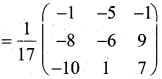substituting in (1)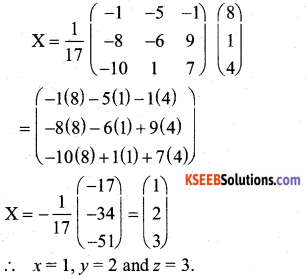Question 42.
If y = 3 cos (logex) + 4 sin (logex) show that x2y2 + xy1 + y = 0.
y = 3 cos (logex) + 4sin (logex)
Diff wrt x
y1 = 3(-sin(logx).$$\frac { 1 }{ x }$$) + 4 cos (log x). $$\frac { 1 }{ 2 }$$
xy1 = -3 sin (logx) + 4 cos(logx)
diff wrt x
xy2 y1. 1 = -3cos(logx).$$\frac { 1 }{ x }$$ + 4(-sin(logx)).$$\frac { 1 }{ x }$$
xy2 + y1 = $$\frac { 1 }{ 2 }$$-(3cos(logx) + 4sin(logx))
x2y2 + xy1 = -(3cos(logx) + 4sin(logx))
x2y2 + xy1 = -y
∴ x2y2+ xyj1+ y = 0

Question 43.
Sand is pouring from a pipe at the rate of 12cm3/sec. The falling sand forms a cone on the ground in such a way that the height of the cone is always one – sixth of the radius of the base. How fast is the height of the sand cone increasing when the height is 4 cm?
Volume, V = $$\frac{\pi r^{2} h}{3}$$
given; h = $$\frac { 1 }{ 6 }$$ r (or) r = 6h
∴ v = $$\frac{\pi}{3}$$ × (6h)2 ×h = 12πh3
v = 12πh3
diff w.r.t ‘t’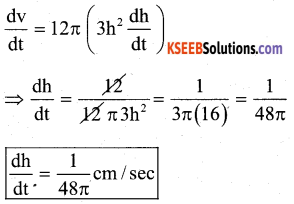Question 44.
Find the integral of $$\sqrt{x^{2}+a^{2}}$$ with respect to x, and hence find $$\int \sqrt{x^{2}+2 x+5} d$$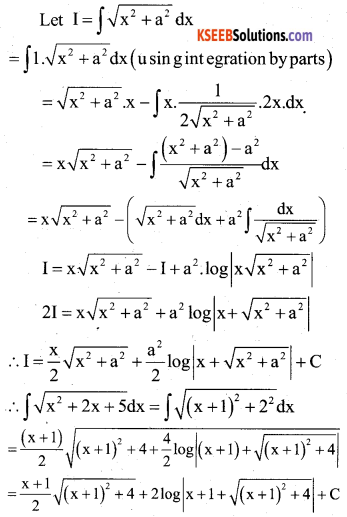Question 45.
Using integration find the area of the region in the first quadrant enclosed by the x – axis, the line y = x, ad circle x2 + y2 = 32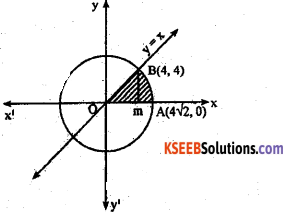x2 + y2 = 32 and centre (0,0)
∴ radius = r = $$\sqrt{32}$$ = $$4 \sqrt{2}$$
Equation are y = x ….(1) and x2 + y2 = 32 …..(2)
∴x2 + x2 = 32 (usinig (1) and (2))
∴ 2x2 = 32 ⇒ x2 = 16 or x = ±
since region is in first quadrant
x = 4 ⇒ y = 4
∴ Required Area = Area OBM + Area MBA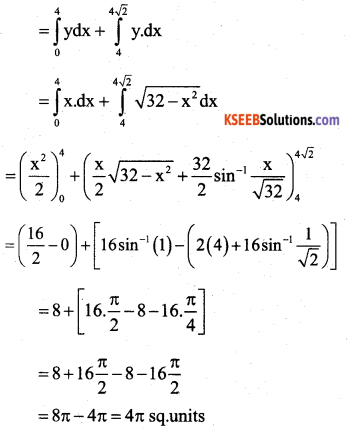Question 46.
Find the general solution of the differential equation (x+ y)$$\frac{d y}{d x}$$ = 1
(x + y)$$\frac{d y}{d x}$$ = l dx
(x + y)dy = dx or $$\frac{d y}{d x}$$ = x + y
∴ $$\frac{d y}{d x}$$ -x = y which i s in the form
$$\frac{d y}{d x}$$ + Px =Q dy
Here P = -1; Q = y
∴ Integrating Factor = IF = $$e^{\int P d y}$$
$$=e^{\int(-1) d y}=e^{-y}$$
∴ General solution is
x(IF) = $$\int y \cdot(I F) d y+C$$ (Using integration by parts)
= y(-e-y) – $$\int-\left(e^{-y}\right)$$.1.dy + C
= -ye-y +$$\int e^{-y} d y$$ + C ÷ e-y
xe-y = -ye-y – e-y + C
⇒ x = -y-l+C.ey
Or x + y + 1 = C.eyQuestion 47.
Derive the equation of a plane in normal form both in the vector and Cartesian form.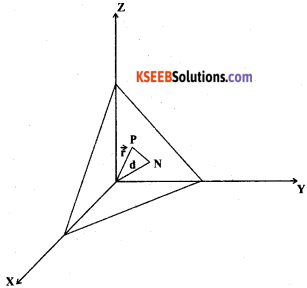Consider a plane whose perpendicular distance from the origin is d. where d ≠ 0.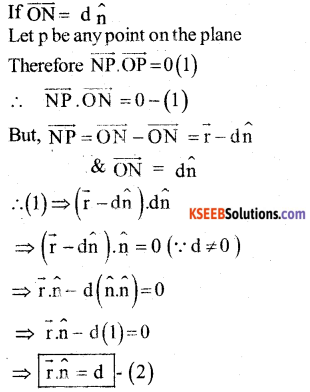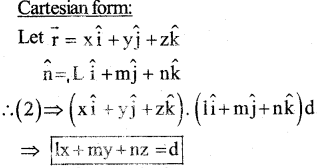This is the Required Equation of a plane m normal form.
To find P and Q :
x2 = 4y and x =4y – 2
=>(4y – 2)2 = 4y ⇒ 16y2 + 4 – 16 y=4 y
⇒ 16y2 – 20y +4 = 0
⇒ (y – 1) (16y – 4) = 0
⇒ y = 1 (or) y = $$\frac { 1 }{ 4 }$$
if y = 1 ; x = 2 ⇒ 2 (2,1)
and y = $$\frac { 1 }{ 4 }$$ ; x = -1 ⇒ (-1, $$\frac { 1 }{ 4 }$$)
Thus the points of intersection are
(2,1) I(-1 .$$\frac { 1 }{ 4 }$$)
∴ Area of the Region O B Q C = Area of BCQ + Area of QCO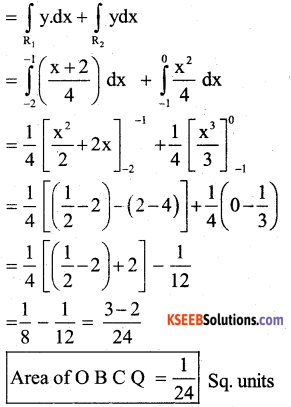Question 48.
A die is thrown 6 times. If ‘getting an odd number’ is success, what is the probability of
a) 5 successes
b) at least 5 successes
c) at most 5 Successes
Let P = probability of getting an odd number = $$\frac { 3 }{ 6 }$$ = $$\frac { 1 }{ 2 }$$
q = 1 – p = 1$$\frac { -1 }{ 2 }$$ = $$\frac { 1 }{ 2 }$$
& n =6
we have P(x) = ncx Px qn-x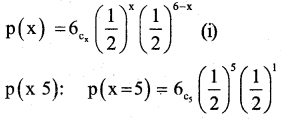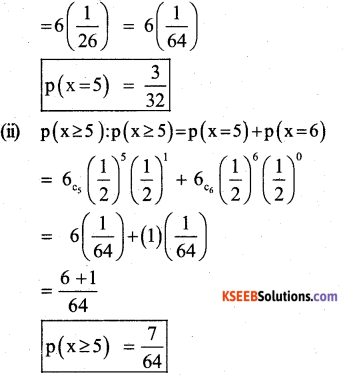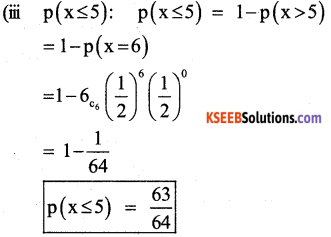Part-E

Answer any ONE question: (1 × 10 = 10)

Question 49.
a) Prove that $$\int_{0}^{b} f(x) \cdot d x=\int_{a}^{b} f(a+b-x) d x$$ and hence. Evaluate $$\int_{\pi / 3}^{\pi / 3} \frac{1}{1+\sqrt{\tan x}} d x$$ (June – 2014,Q.No.49a)
Solution :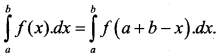let t = a + b – x then dt = -dx
when x =a and x = b
t = b t = a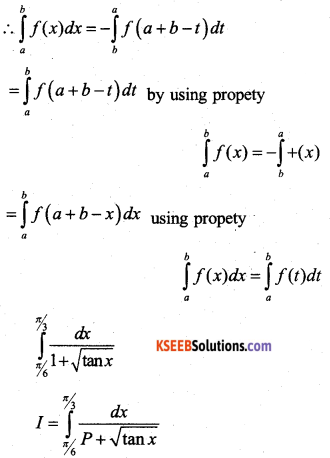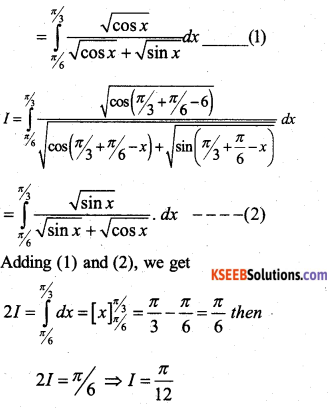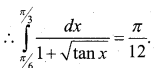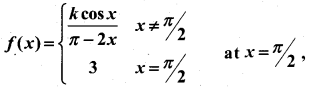f(x) is continuous, find the value of k.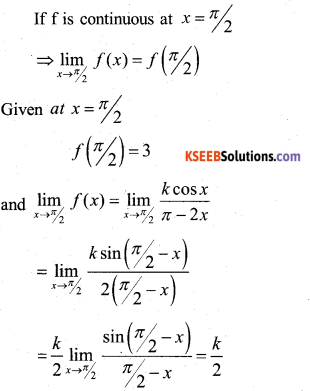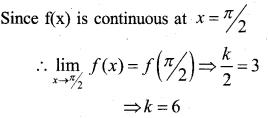Question 50.
(a) A factory manufactures two types of screws, A and B. Each type of Screw requires the use of two machines, an automatic and a hand operated. It takes 4 minutes on the automatic and 6 minutes on hand operated machines to manufacture a package of screws A, while it takes 6 minutes on automatic and 3 minutes on the hand operated machines to manufacture a package of screws B. Each machine is available for at most 4 hours on any day. The manufacture can sell a package of screws A at a profit of Rs.7 and seres B at a profit of Rs.10. Assuming that he can sell all the screws he manufactures, how many packages of each type should the factory owner produce in a day in order to maximise his profit? Determine the maximum profit.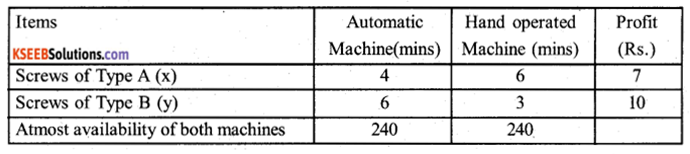∴ Maximise Z = 7x + 10y subject to constraints
4x + 6y ≤ 240
or 2x + 3y ≤ 120 …… (1)
and 6x + 3y ≤ 240
or 2x + y ≤ 80 …… (2)
and x, y ≥ 0
Converting inequalities to equalities
2x + 3y= 120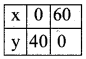2x + y = 80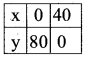Plot the graph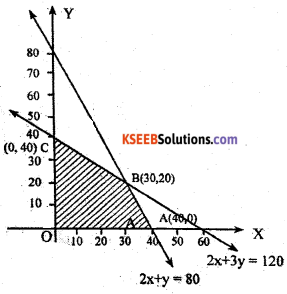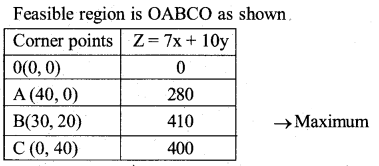Maximum profit is Rs.410 which occurs at point B (30, 20)
∴Manufacturer should manufacture 30 packages of screws A and 20 packages of screws B.